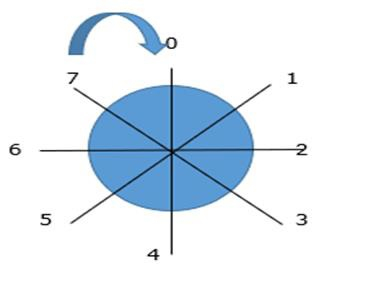# Explain bit field in C language by using structure concept

Bit field is used for specifying the size of variable in terms of bits. Generally, it is defined inside a structure.

• Bit field: 1 byte=8 bits

For example,

An example is explained below −

Struct info{
int x:2;
};

Here, x is occupying 2bits.

It is invalid to assign any value to a bit field out of its range.

We cannot use scanf statements to input the value of bit field because, size of and address operator cannot apply to bit field.

The data types that we can assign to a bit field can be int, signed int, unsigned int.

## Program

Following is the C program for unsigned int

Live Demo

#include<stdio.h>
struct info{
unsigned int x:3;// assign bit field to unsigned int inside structure
};
main(){
struct info i;
i.x=8;
printf("%d",i.x);
}

## Output

The output is as follows &miuns;

0

## Explanation

• Range formula for unsigned int is 0 to 2n-1 and n=no of bits.

• Here, n=3, i.e., unsigned int is in between 0 to 23 -1, which is equal to 0 to 7.## Program

Refer the program given below for int

Live Demo

#include<stdio.h>
struct info{
int x:3;// assign bit field to int inside structure
};
main(){
struct info i;
i.x=4;
printf("%d",i.x);
}

## Output

You will get the following output −

-4

## Explanation

• Range formula for signed int = (-2(n-1)+1) to 2n-1, where n is no of bits.
• In the program, n=3
• Substitute this in the formula and we have the following result −
=(-2(3-1)+1) to 23-1
=(-22+1) to 22
= -3 to 4 i.e., -3,-2,-1,0,1,2,3,4,